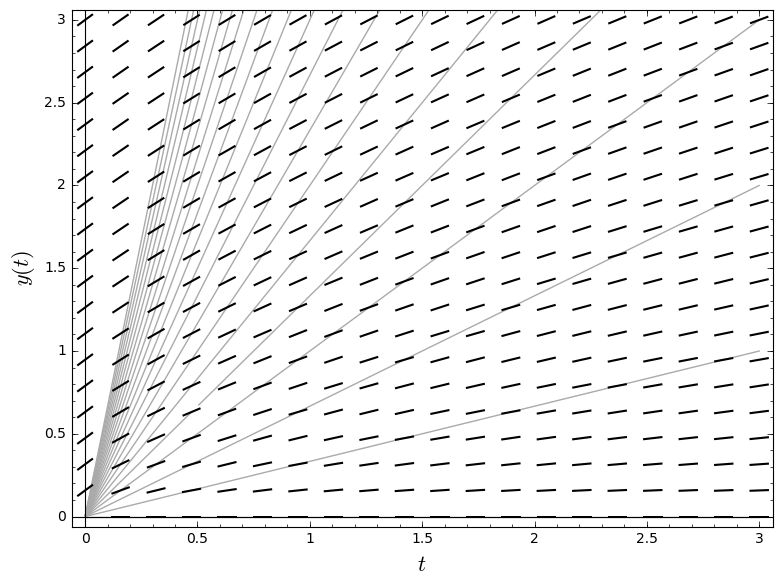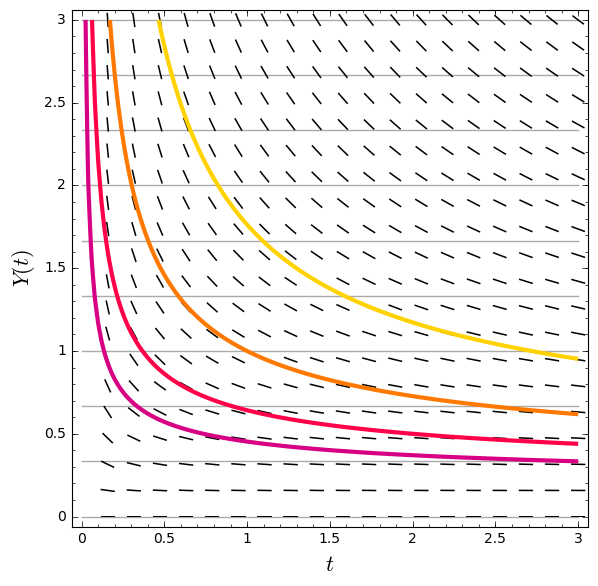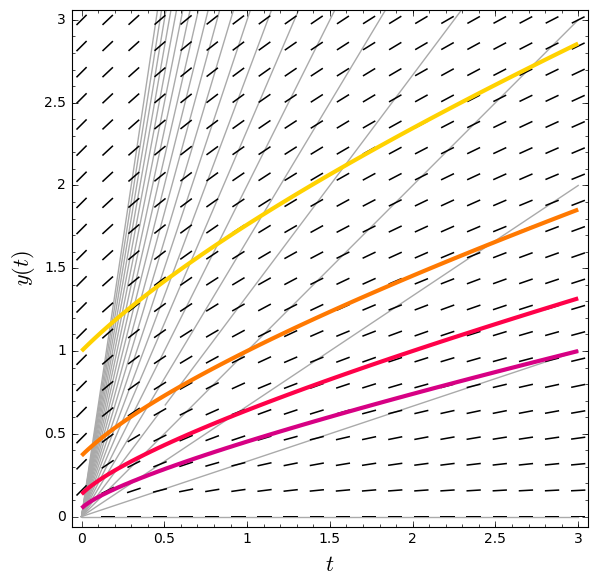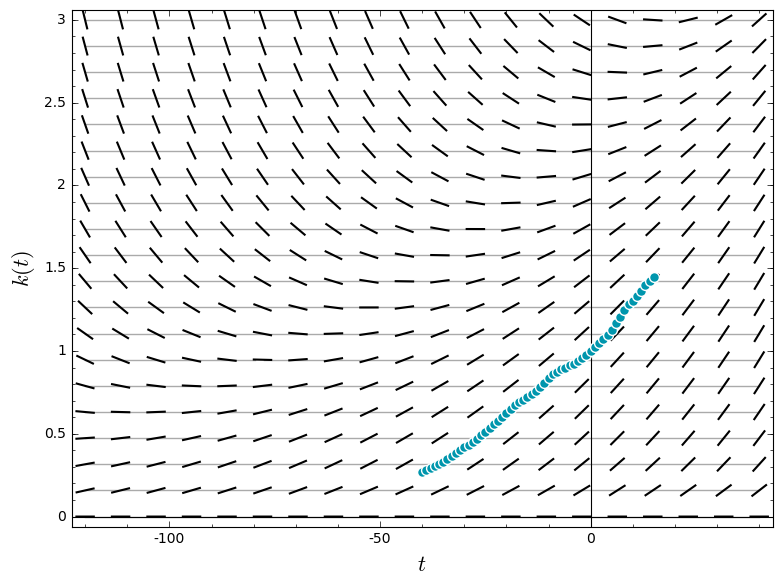# Other rescalings¶

In :
t, y, Y, k, m, M = var('t y Y k m M')
palette = [(215/255, 0/255, 132/255), (255/255, 1/255, 73/255), (255/255, 121/255, 1/255), (255/255, 210/255, 0/255)]
cool_palette = [(0/255, 150/255, 173/255), (0/255, 200/255, 146/255)]
grid_gray = (2/3, 2/3, 2/3)


## A (projective) homogeneous equation¶

\begin{align*} y' & = \frac{y}{t + y} = \frac{y/t}{1 + y/t} \\ Y(t) & = y(t)/t \\ Y' & = y'/t - y/t^2 \\ & = (y' - Y)/t \\ & = \left(\frac{Y}{1 + Y} - Y\right)\frac{1}{t} \\ & = \left(\frac{-Y^2}{1 + Y}\right)\frac{1}{t} \\ \left(\frac{1}{Y^2} + \frac{1}{Y}\right)Y' & = -\frac{1}{t} \end{align*}

In :
homog_grid = sum([plot(n/3 * t, (t, 0, 3), ymax = 3, rgbcolor = grid_gray, zorder = 0) for n in range(21)])
homog_direction = plot_slope_field(y/(t + y), (t, 0, 3), (y, 0, 3))
homog_labels = ["$t$", "$y(t)$"]
show(homog_direction + homog_grid, axes_labels = homog_labels)In :
scaled_homog_grid = sum([plot(n/3, (t, 0, 3), rgbcolor = grid_gray, zorder = 0) for n in range(10)])
scaled_homog_direction = plot_slope_field(-Y^2/(1 + Y) * (1/t), (t, 0, 3), (Y, 0, 3))
scaled_homog_solutions = [implicit_plot(-1/Y + log(Y*t) + (3-n), (t, 0, 3), (Y, 0, 3), linewidth = 3, color = palette[n]) for n in range(4)]
scaled_homog_labels = ["$t$", "$Y(t)$"]
show(scaled_homog_direction + scaled_homog_grid + sum(scaled_homog_solutions), axes_labels = scaled_homog_labels)In :
homog_solutions = [implicit_plot(-t/y + log(y) + (3-n), (t, 0, 3), (y, 0, 3), linewidth = 3, color = palette[n]) for n in range(4)]
show(homog_direction + homog_grid + sum(homog_solutions), axes_labels = homog_labels)## Economic growth¶

One way to measure the size of an economy is to look at its stock of capital—the stuff you use to make more stuff. Let's say $k(t)$ is the amount of capital in Canada $t$ years after the year 2000. In the "Solow-Swan model" of economic growth, an economy produces new capital at a rate that depends on the amount of capital and the amount of labor available. Let's assume the amount of labor is proportional the population, $l(t) = e^{t/80}$. Old capital wears out at a rate proportional to the amount of capital. Overall, the stock of capital changes something like this:

\begin{align*} k'(t) & = \tfrac{1}{8} l(t)^{1/4} k(t)^{3/4} - \tfrac{1}{10} k(t) \\ & = \tfrac{1}{8} e^{t/320}\,k(t)^{3/4} - \tfrac{1}{10} k(t). \end{align*}

This is an example of a "Bernoulli equation."

In :
capital_grid = sum([plot((3/19)*n, (t, -120, 40), rgbcolor = grid_gray, zorder = 0) for n in range(20)])
capital_direction = plot_slope_field((1/8)*exp(t/320)*k^(3/4) - (1/10)*k, (t, -120, 40), (k, 1e-6, 3))
capital_labels = ["$t$", "$k(t)$"]
show(capital_direction + capital_grid + capital_scatter, axes_labels = capital_labels)You can solve a general Bernoulli equation

$$k'(t) = p(t)\,k(t) + q(t)\,k(t)^\alpha$$

by looking at a new unknown function $m(t) = k(t)^{1-\alpha}$. In our case, $m(t) = k(t)^{1/4}$, and

$$4 m'(t) = \tfrac{1}{8} e^{t/320} - \tfrac{1}{10} m(t).$$

In :
squashed_capital_grid = sum([plot(((3/19)*n)^(1/4), (t, -120, 40), rgbcolor = grid_gray, zorder = 0) for n in range(1.5^4*19/3)])
squashed_capital_direction = plot_slope_field((1/32)*exp(t/320) - (1/40)*m, (t, -120, 40), (m, 1e-6, 1.5))
squashed_capital_labels = ["$t$", "$m(t)$"]
show(squashed_capital_direction + squashed_capital_grid, axes_labels = squashed_capital_labels)